#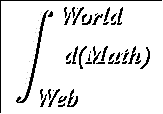The Quotient Rule

Suggested prerequestites: Definition of the derivative, The Product Rule

Now that we've seen how the derivative of a product is found, we can extend the method to quotients. In fact, after the direct approach, we'll show how the quotient rule may be obtained from the product rule with only a little sleight of hand.

To begin the direct approach, we apply the definition of the derivative to the ratio u(x)/v(x) in order to find a general formula;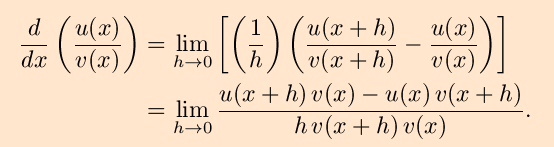We again use the trick of adding zero, this time in the form of -u(x)v(x) +u(x)v(x) to obtain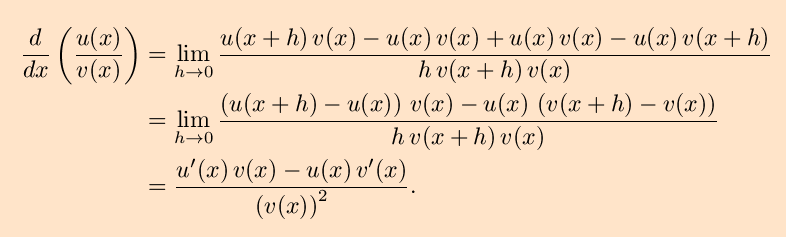So, the quotient rule for differentiation is ``the derivative of the first times the second minus the first times the derivative of the second over the second squared.'' Quite a mouthful but still useful.

You might note that the above is far from rigorous, in that we used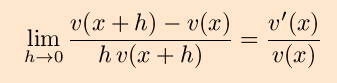and a similar limit for u´(x)/v(x). However, an implicit assumption in this derivation is that v(x) is (a) non-zero at the points where the derivative is evaluated and (b) differentiable, and hence continuous at these points. In any event, the resultis valid whenever u´(x) and v(x) are differentiable and v(x) is nonzero, and may be used with impunity.
We can use the quotient rule to find the derivative of x-n where n is a positive integer, by writing the expression instead as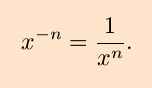Application of the quotient rule then gives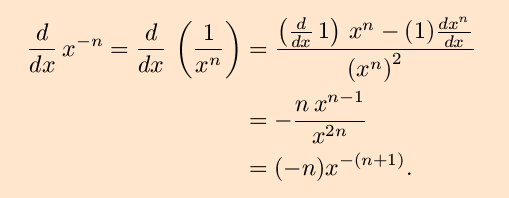This is what we would would have gotten by applying the power rule without considering the sign of the power. By this, then, we know that the power rule,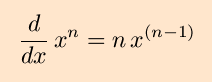can and should be extended to all integers.

To see how the quotient rule is obtained from the product rule, define a function w(x) by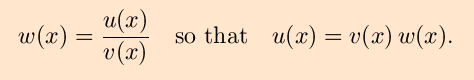Now, apply the product rule to u(x) (the x-dependence will be supressed) and solve for w´;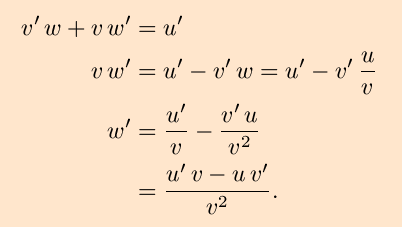# Exercises:

Find the derivatives of the following functions.

1.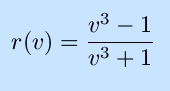2.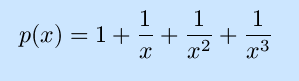3.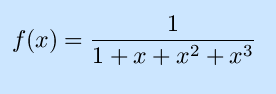Solutions to the exercises | Back to the Calculus page | Back to the World Web Math top page

watko@mit.edu